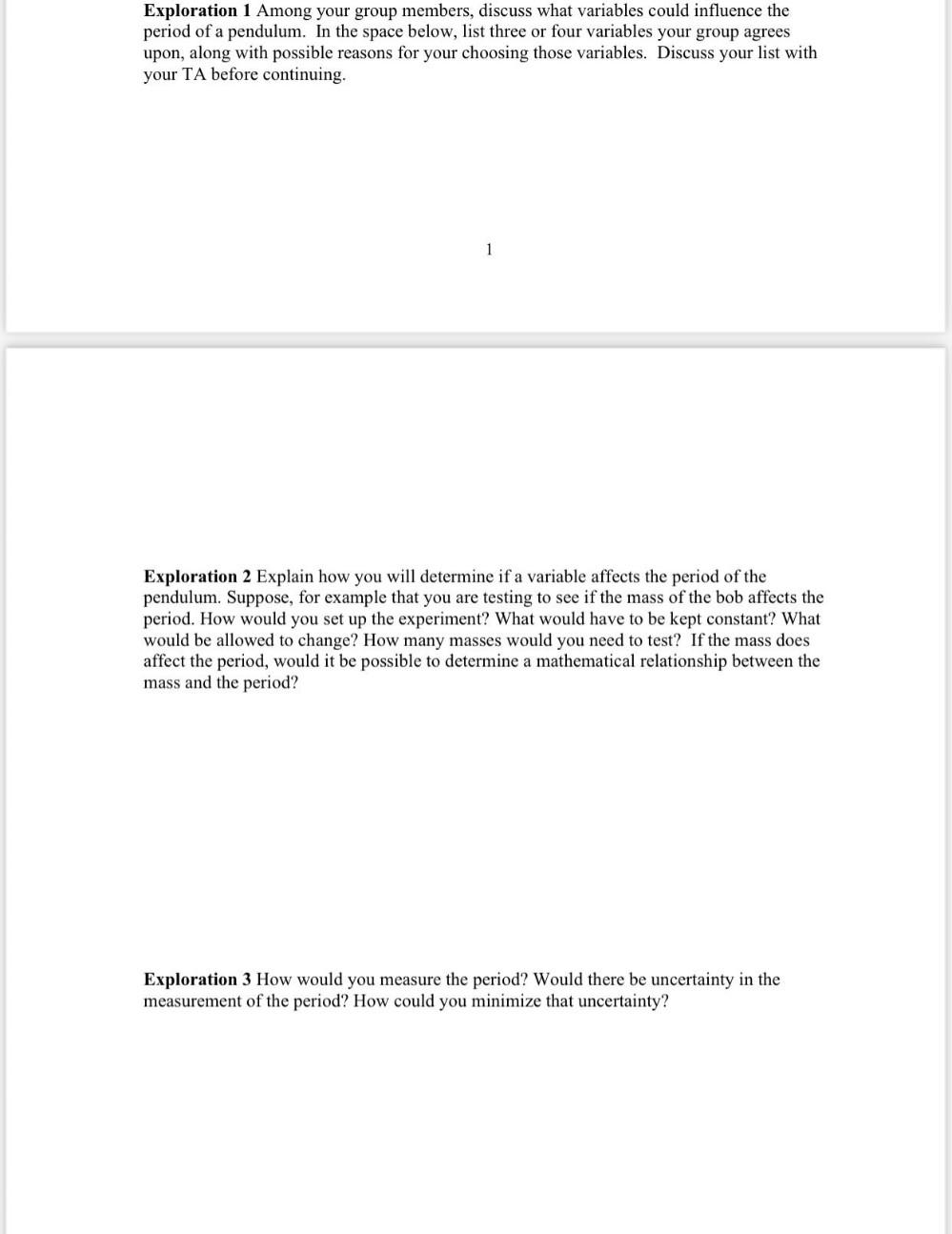Question:

# Exploration 1 Among your group members, discuss what variables could influence the period of a pendulum. In the space below, lisExploration 1 Among your group members, discuss what variables could influence the period of a pendulum. In the space below, list three or four variables your group agrees upon, along with possible reasons for your choosing those variables. Discuss your list with your TA before continuing. 1 Exploration 2 Explain how you will determine if a variable affects the period of the pendulum. Suppose, for example that you are testing to see if the mass of the bob affects the period. How would you set up the experiment? What would have to be kept constant? What would be allowed to change? How many masses would you need to test? If the mass does affect the period, would it be possible to determine a mathematical relationship between the mass and the period? Exploration 3 How would you measure the period? Would there be uncertainty in the measurement of the period? How could you minimize that uncertainty?